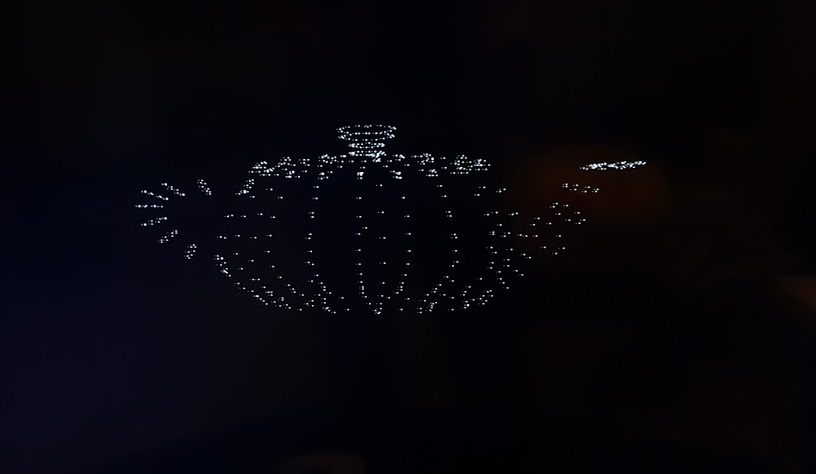# GPU Project 08 – Point cloud rendering in FPGAAfter successfully running the simulation, its time to see how the rendering works on the real HW. And as always, SW needs to be written to get the HW to know what to do. In this case, I will be loading the vertex data of a cube into memory to see it transformed, and then experiment with the same teapot data that I used in the C# version the app.

So, first off I created a little C function that adds the 8 vertices of the cube in any location in space:

``````void create_cube( float size, float o_x, float o_y, float o_z ){
add_vertex( o_x + 0, o_y + 0, o_z + 0);
add_vertex( o_x + 1, o_y + 0, o_z + 0);
add_vertex( o_x + 0, o_y + 1, o_z + 0);
add_vertex( o_x + 1, o_y + 1, o_z + 0);
add_vertex( o_x + 0, o_y + 0, o_z + 1);
add_vertex( o_x + 1, o_y + 0, o_z + 1);
add_vertex( o_x + 0, o_y + 1, o_z + 1);
add_vertex( o_x + 1, o_y + 1, o_z + 1);
num_objects++;
}
``````

The vertices are added as a list directly into the BRAM vertice memory block:

```void add_vertex(float x, float y, float z){
reg_write(local_vertex_buffer, num_vertexes *16 + 0, float2fixedspace(x) );
reg_write(local_vertex_buffer, num_vertexes *16 + 4, float2fixedspace(y) );
reg_write(local_vertex_buffer, num_vertexes *16 + 8, float2fixedspace(z) );
num_vertexes++;
}
```

And when its time to render the scene, we just tell the main register bank how many vertices are in the system:

``````void gpu_render(uint32_t vertexes){
reg_write(localgpu, 0x10, vertexes);	// Number of vertexes
reg_write(localgpu, 0x00, 1);			// Start
}
``````

Along with the rest of the VDMA code we already had, this is what we get on the HDMI port:Not very spectacular.. but this is about the simplest object we can show. After playing a while and exporting the dataset as an array that I could load as a header file:Looks like we are on track!